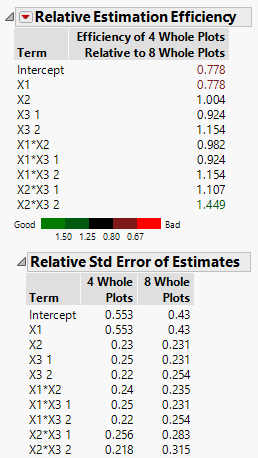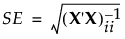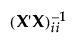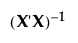Publication date: 03/23/2021

## Relative Estimation Efficiency

The Relative Estimation Efficiency report compares designs in terms of the standard errors of parameter estimates for parameters in the assumed model. The standard errors control the length of confidence intervals for the parameter estimates. This report provides an efficiency ratio and the relative standard errors.

The relative estimation efficiency values are colored according to a color gradient shown under the table of relative estimation efficiency values. You can control the color legend using the options in the Relative Estimation Efficiency red triangle menu. See Color Dashboard.

Figure 16.26 Relative Estimation Efficiency Comparing Two Split-Plot DesignsFigure 16.26 shows the Relative Estimation Efficiency outline for the split-plot designs compared in Split Plot Designs with Different Numbers of Whole Plots.

### Relative Estimation Efficiency

For a given term, the estimation efficiency of the reference design relative to a comparison design is the relative standard error of the term for the comparison design divided by the relative standard error of the term for the reference design. A value less than one indicates that the reference design is not as efficient as the comparison design. A value greater than one indicates that it is more efficient.

### Relative Standard Error of Estimates

The Relative Std Error of Estimates report gives the ratio of the standard deviation of a parameter’s estimate to the error standard deviation. These values indicate how large the standard errors of the model’s parameter estimates are, relative to the error standard deviation. For the ith parameter estimate, the Relative Std Error of Estimate is defined as follows:where:

X is the model matrix defined in The Alias Matrix, andis the ith diagonal entry of.

Want more information? Have questions? Get answers in the JMP User Community (community.jmp.com).